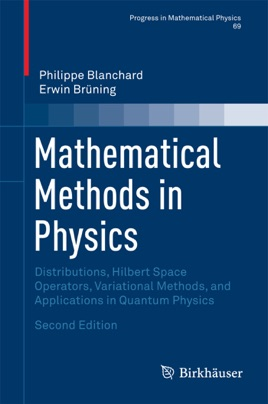• 87,99 €

## Publisher Description

The second edition of this textbook presents the basic mathematical knowledge and skills that are needed for courses on modern theoretical physics, such as those on quantum mechanics, classical and quantum field theory, and related areas.  The authors stress that learning mathematical physics is not a passive process and include numerous detailed proofs, examples, and over 200 exercises, as well as hints linking mathematical concepts and results to the relevant physical concepts and theories.  All of the material from the first edition has been updated, and five new chapters have been added on such topics as distributions, Hilbert space operators, and variational methods.

The text is divided into three main parts. Part I is a brief introduction to distribution theory, in which elements from the theories of ultradistributions and hyperfunctions are considered in addition to some deeper results for Schwartz distributions, thus providing a comprehensive introduction to the theory of generalized functions. Part II contains fundamental facts about Hilbert spaces and their geometry. The theory of linear operators, both bounded and unbounded, is developed, focusing on results needed for the theory of Schrödinger operators. Part III treats the direct methods of the calculus of variations and their applications to boundary- and eigenvalue-problems for linear and nonlinear partial differential operators. The appendices contain proofs of more general and deeper results, including completions, basic facts about metrizable Hausdorff locally convex topological vector spaces, Baire's fundamental results and their main consequences, and bilinear functionals.

Mathematical Methods in Physics is aimed at a broad community of graduate students in mathematics, mathematical physics, quantum information theory, physics and engineering, as well as researchers in these disciplines. Expanded content and relevant updates will make this new edition a valuable resource for those working in these disciplines.